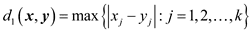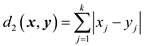# Concept behind equivalent metrics

• I
My professor never really went over this concept clearly in Advanced Calculus.
The definition of an equivalent metric from my lecture slides:
Two metrics: d1 and d2 on a metric space X are called equivalent when there exists M >= 1 such that M-1d1(x,y) <= d2(x,y) <= Md2(x,y)

I am trying to prove this question
Show that the properties “{xn} converges to x”, “x is a cluster point of {xn}”, “{xn} is Cauchy” and “X is complete”, each remain unchanged when the metric is replaced by an equivalent metric.

However, I need to first understand the meaning behind equivalent metrics before trying to solve the problem and i've been spending an hour on it and still I don't get it. Please explain the concept behind equivalent metrics. If possible, try setting up diagrams of open/closed balls and what not to try to better understand the concept.

fresh_42
Mentor
My professor never really went over this concept clearly in Advanced Calculus.
The definition of an equivalent metric from my lecture slides:
Two metrics: d1 and d2 on a metric space X are called equivalent when there exists M >= 1 such that M-1d1(x,y) <= d2(x,y) <= Md2(x,y)

I am trying to prove this question
Show that the properties “{xn} converges to x”, “x is a cluster point of {xn}”, “{xn} is Cauchy” and “X is complete”, each remain unchanged when the metric is replaced by an equivalent metric.

However, I need to first understand the meaning behind equivalent metrics before trying to solve the problem and i've been spending an hour on it and still I don't get it. Please explain the concept behind equivalent metrics. If possible, try setting up diagrams of open/closed balls and what not to try to better understand the concept.
It's simply a scaling factor, just as if you changed from a ruler with inches to one with centimeters. The properties you mentioned don't depend on a constant factor.

member 587159
There is really not much to add besides the definition. Maybe an instructive exercise for you can help:

Show that ##\Vert \cdot \Vert_1, \Vert \cdot \Vert_2## and ##\Vert \cdot \Vert_\infty## are equivalent metrics on ##\mathbb{R}^n##.

WWGD
Gold Member
The two give rise to the same topology, i.e., every set open under one topology is open in the other. You may want to show or see a proof of the fact that in a finite-dimensional normed space, any two norms are equivalent. There was a nice proof in Planet Math a while back. EDIT: Try showing that the condition of metric equivalence implies this. You may also try to see what happens with the different "chains" of topologies on a fixed space X , i.e., two topologies T_1X, T_2X are related if collection of open sets in T_1X is a subset of collection of open sets in T_2X. and see which properties are preserved in different cases.

mathwonk
Homework Helper
2020 Award
look at a convergent sequence in a metric space. now step back a few paces. it should still look convergent. hence equivalent (i.e. scaled) metrics do not change convergence.

member 587159
The two give rise to the same topology, i.e., every set open under one topology is open in the other. You may want to show or see a proof of the fact that in a finite-dimensional normed space, any two norms are equivalent. There was a nice proof in Planet Math a while back. EDIT: Try showing that the condition of metric equivalence implies this. You may also try to see what happens with the different "chains" of topologies on a fixed space X , i.e., two topologies T_1X, T_2X are related if collection of open sets in T_1X is a subset of collection of open sets in T_2X. and see which properties are preserved in different cases.

I doubt that the OP who just learns about metric spaces and equivalent metrics knows what a topology is, but I might be wrong.

WWGD
Gold Member
I doubt that the OP who just learns about metric spaces and equivalent metrics knows what a topology is, but I might be wrong.
You're right, let's see, I may have jumped the gun.

I doubt that the OP who just learns about metric spaces and equivalent metrics knows what a topology is, but I might be wrong.
Well i'm taking Advanced Calculus 1, so it's only topological concepts of metric spaces and not a whole lot on topological spaces in general.

Ok, here is one example. Take two metrics d1 and d2Would these two metrics be equivalent?

#### Attachments

fresh_42
Mentor
Ok, here is one example. Take two metrics d1 and d2
View attachment 222857
View attachment 222858
Would these two metrics be equivalent?
How do ##\max\,|x_i-y_i|## and ##\sum_i\,|x_i-y_i|## relate? You should use the finite dimension here.

mathwonk
Homework Helper
2020 Award
obviously i am delusional but i thought my post #5 nailed it. ie just because the points look twice as close together, they are still either convergent or not.

convergence means the distances go to zero, so doubling those distances or halving them does nothing to this conclusion.

•jim mcnamara
Erland
Two metrics: d1 and d2 on a metric space X are called equivalent when there exists M >= 1 such that M-1d1(x,y) <= d2(x,y) <= Md2(x,y)
There is a typo here, which perhaps causes trouble. The last Md2(x,y) should be Md1(x,y),
so the correct inequalities are:
M-1d1(x,y) <= d2(x,y) <= Md1(x,y)

•lavinia
My professor never really went over this concept clearly in Advanced Calculus.
The definition of an equivalent metric from my lecture slides:
Two metrics: d1 and d2 on a metric space X are called equivalent when there exists M >= 1 such that M-1d1(x,y) <= d2(x,y) <= Md2(x,y)
That definition corresponds to strongly equivalent metrics. Two metrics can be equivalent without being strongly equivalent.
Equivalent in this case means that they give rise to the same topology

fresh_42
Mentor
Two metrics can be equivalent without being strongly equivalent.
Can you elaborate this?

Can you elaborate this?

Let d(x,y) be an unbounded metric then the metric is equivalent to
$$\overline{d}(x,y)=Min\{d(x,y),1\}$$
and they are not strongly equivalent for there is no C>0 such that ##d(x,y)\leq C \overline{d}(x,y)##

•FactChecker, WWGD and lavinia
lavinia
Gold Member
Ok, here is one example. Take two metrics d1 and d2
View attachment 222857
View attachment 222858
Would these two metrics be equivalent?

Note that in post #12 Erland pointed out a typo in the original post and has corrected it.

These metrics are equivalent.

However they are not scalings of each other, i.e. a mere change of units. For instance take ##k=2## and consider the points of distance 1 to the origin. In the max metric, this is the unit square, ##S_1##,all points with coordinates ##(x,1)## or ##(1,y)## with ##|x|## and ##|y|## less than or equal to 1.

In the sum of the coordinate distances metric, points of distance one satisfy ##|x|+|y|=1##. This is also a square ,##S_2##, but the sides connect the points ##(1,0)## and ##(-1,0)## to the points ##(0,1)## and ##(0,-1)##. There is no way to scale one of these squares to become the other since they are rotated by 90 degrees with respect to each other.

Note that ##S_1## lies completely outside of ##S_2## so its ##d_2## distance to the origin is bigger than 1( which is also obvious from the definitions of the two metrics. ). If ##S_1## is shrunk by a scale factor of 1/2 then it lies completely inside of ##S_2## so all of the scaled points have ##d_2## metric less than or equal to 1 (with its four corners exactly equal to 1). This tells you the ##M## for these two equivalent metrics.

Both of these metrics are equivalent to the usual Pythagorean metric. But a square cannot be scaled into a circle.

Last edited:
lavinia
Gold Member
My professor never really went over this concept clearly in Advanced Calculus.
The definition of an equivalent metric from my lecture slides:
Two metrics: d1 and d2 on a metric space X are called equivalent when there exists M >= 1 such that M-1d1(x,y) <= d2(x,y) <= Md2(x,y)

However, I need to first understand the meaning behind equivalent metrics before trying to solve the problem and i've been spending an hour on it and still I don't get it. Please explain the concept behind equivalent metrics. If possible, try setting up diagrams of open/closed balls and what not to try to better understand the concept.

I always thought that metrics are equivalent if every open set in one is open in the other. Open means that around any point in the set there is an open ball completely contained within the set. This is the same as saying that every Cauchy sequence in one is a Cauchy sequence in the other.

The definition that you were given certainly implies that the metrics have the same open sets. But the cool example in post #15 shows that one can have the same open sets even when the two inequalities are not both satisfied.

mathwonk